Next: Image Formation Model Up: tr05mj1 Previous: Introduction

# Problem Formulation

Consider an image,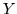, generated by some image generation process,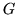, from a known (ground truth) object,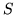, whereis not spatially aligned with, but related spatially by a transformation,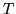. The objective of the segmentation/registration problem is to recover the spatial transformation,, which relatesto. That is, findsuch that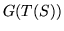andare most similar'.

A Bayesian formulation of this problem is as follows:

•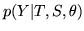is the image formation/likelihood model where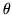are parameters of the generation process (e.g. noise variance, tissue intensities).
•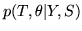is the joint posterior probability distribution for the spatial transformation (the quantity of interest) and the unknown generation parameters.
•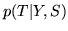is the marginal posterior distribution, which integrates over the unknown parameters,.

Note that generallyis parameterised by its own set of parameters, and thatis giving the posterior probability for these transformation parameters.

The probabilities are related by: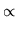(1)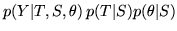(2)(3)

and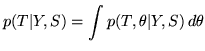(4)

where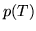is the prior probability distribution for the transformation,, and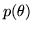is the prior for the image formation parameters,. It is assumed thatandare independent, so that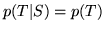. Similarly forand. The constant of proportionality,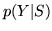, does not depend onand hence will be ignored for the remainder of this report.

Note that marginalising overis the difficult step in calculating.

The problem of finding the best' single segmentation/registration1 is then equivalent to finding the maximum a-posterior probability: the MAP estimate.

That is: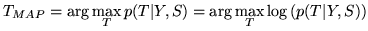Therefore, or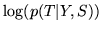, play an equivalent role to the similarity function in common registration techniques.

SubsectionsNext: Image Formation Model Up: tr05mj1 Previous: Introduction